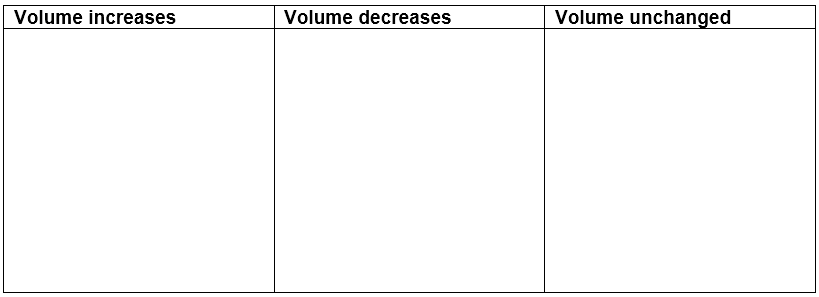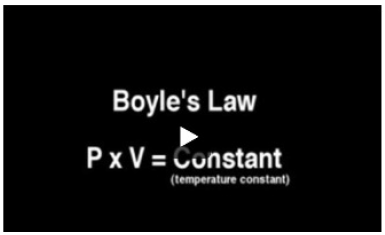# Problem: Standard temperature and pressure (STP) are considered to be 273 K and 1.0 atm. Predict which of the following changes will cause the volume of the balloon to increase or decrease assuming that the temperature and the gas filling the balloon remain unchanged.Balloon filled with helium at STP floats into the atmosphere where the pressure is 0.5 atm.Balloon filled with helium at STP is submerged under water where the pressure if 1.25 atm.Balloon filled with helium at STP floats into air where the pressure equals 1 atm.Balloon filled with helium under water at 1.15 atm is released and floats to the surface, which is at STP.Watch the video that shows the relationship between pressure and volume for a particular gas. Robert Boyle was the first one to observe the change in the pressure of a gas when its volume is increased or decreased at a constant temperature and the change in the volume of a gas when its pressure is increased or decreased at a constant temperature.

###### FREE Expert Solution
91% (304 ratings)View Complete Written Solution
###### Problem Details

Standard temperature and pressure (STP) are considered to be 273 K and 1.0 atm. Predict which of the following changes will cause the volume of the balloon to increase or decrease assuming that the temperature and the gas filling the balloon remain unchanged.Balloon filled with helium at STP floats into the atmosphere where the pressure is 0.5 atm.

Balloon filled with helium at STP is submerged under water where the pressure if 1.25 atm.

Balloon filled with helium at STP floats into air where the pressure equals 1 atm.

Balloon filled with helium under water at 1.15 atm is released and floats to the surface, which is at STP.

Watch the video that shows the relationship between pressure and volume for a particular gas. Robert Boyle was the first one to observe the change in the pressure of a gas when its volume is increased or decreased at a constant temperature and the change in the volume of a gas when its pressure is increased or decreased at a constant temperature.Frequently Asked Questions

What scientific concept do you need to know in order to solve this problem?

Our tutors have indicated that to solve this problem you will need to apply the Chemistry Gas Laws concept. You can view video lessons to learn Chemistry Gas Laws. Or if you need more Chemistry Gas Laws practice, you can also practice Chemistry Gas Laws practice problems.

What professor is this problem relevant for?

Based on our data, we think this problem is relevant for Professor Schmidt's class at College of Southern Nevada.